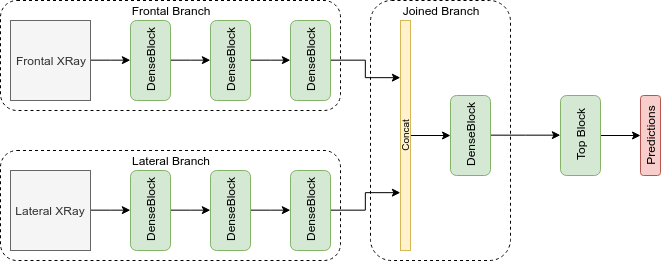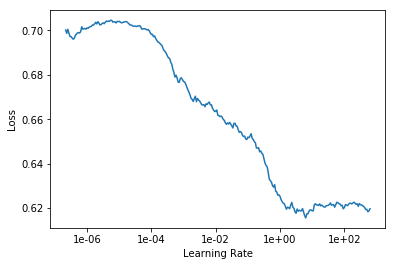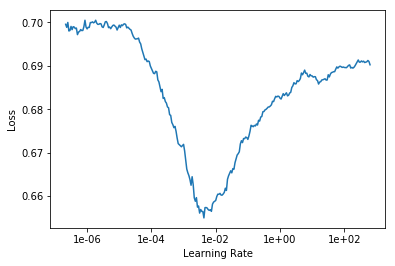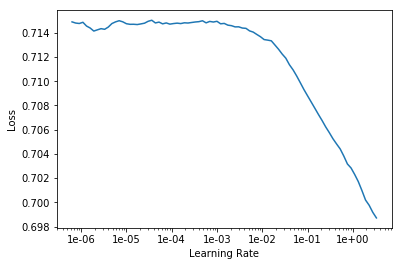# Strange learning rate finder plot for custom model

Hi there!

I am using a custom dual branch architecture (that takes as input a frontal and lateral xray of the same patient) based on DenseNet121. I am using a Learner object and a custom ImageList.

This is how my architecture looks. The frontal and lateral branches are pretrained on ImageNet.This is how I’ve tried to split the model after passing it to the learner:

`learn = learn.split([[learn.model.frontal_cnn, learn.model.lateral_cnn], learn.model.joined_cnn])`

Afterwards I ran the following code to find a good learning rate for my model:

``````learn.freeze()
learn.lr_find(num_it = 300, wd=1e-4, end_lr=1000)
learn.recorder.plot()
``````

However this produces the following weird looking plot. Although I would like it to be true, I don’t thing 1e+2 is a good learning rate for my model.If I unfreeze the whole network and try to find a good learning rate again, (using lr.unfreeze()) the plot looks normal.Am I using the .split() function correctly? And if so, what happened with the first plot?

In these cases, I would rather suggest that 1e-2 and 1e-4 are the best learning rates, respectively. Why don’t you try those values and see if the model trains well?

Thank you very much for the advice! I will use those, but I was wondering about the reason the plot looks so weird and if it somehow indicates a bug somewhere in the pipeline.

I don’t think so. It just mat be some property of your model but it doesn’t necessarily mean there is anything wrong with your model.

I notice that when you call the LR finder you pass in some arguments. Have you tried using the defaults?

I tried using the default arguments:

``````learn.freeze()
learn.lr_find()
learn.recorder.plot()
``````

This generated the following plot:The loss still decreases dramatically at high lr values.

Again a value like lr=0.01 or lr=0.1 is probably fine. I wouldn’t overthink it. As long as the loss is decreasing, your metrics are improving, and your data is not overfitting, you will be fine.